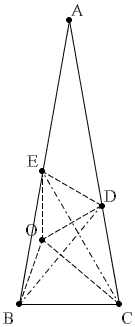# The 80-80-20 Triangle Problem, Solution #6Let ABC be an isosceles triangle (AB = AC) with ∠BAC = 20°. Point D is on side AC such that ∠CBD = 50°. Point E is on side AB such that ∠BCE = 60°. Find the measure of ∠CED.

Solution

This is Solution 5 from [Knop] and is due to Aleksey Borodin, a high school student at the time.

Let O be the circumcenter of ΔBDE.The inscribed angle ∠DBE = 30° and is subtended by the same arc as the central angle DOE. Thus ∠DOE = 60° and ΔDOE is equilateral. In particular, ∠DEO = 60° and EO = DE.

Triangles BDO and BCD are both isosceles implying that CO bisects ∠BCD. Thus ∠DCO = 40°. Since ∠DCE = 20°, CE bisects ∠DCO.

Now, in triangles CDE and COE, EO = ED, CE is a common side and ∠DCE = ∠OCE. We are in a position to claim the equality of the triangles CDE and COE by a non-existent criterion SSA - by two sides and an angle opposite one of them. Usually the SSA does not work as there are two possible angles at E for the given sides and angles at C: one is less, the other greater than 90°. However, in our case, we know from the preceding paragraphs that in both triangles the angles at E are less than 60°, which makes the conclusion valid: ΔCDE = ΔCOE, with the consequence that CE bisects also ∠DEO such that ∠CED = 30°.

### Reference

1. C. Knop, Nine Solutions to One Problem, Kvant, 1993, no 6.### The 80-80-20 Triangle Problem